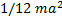# Consider a uniform square of this plate of sideand mass. The moment of inertia of this plate about an axis perpendicular to its plane and passing through one of its corners is a)b)c)d)## Question ID - 53822 :- Consider a uniform square of this plate of sideand mass. The moment of inertia of this plate about an axis perpendicular to its plane and passing through one of its corners is a)b)c)d)3537

(d)

Moment of inertia of square plate aboutMoment of inertia aboutcan be computed using

parallel axis theoremNext Question :
 The radius of a rotating disc is suddenly reduced to half without any change in its mass. Then its angular velocity will be a) Four times b) Double c) Half d) Unchanged